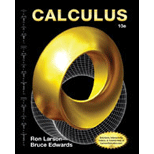Calculus
10th Edition
ISBN: 9781285057095
Author: Ron Larson, Bruce H. Edwards
Publisher: Cengage Learning#### Concept explainers#### Videos

Textbook QuestionChapter 8.8, Problem 82E

Capitalized Cost In Exercises 81 and 82, find the capitalized cost C of an asset (a) for n = 5 years, (b) for n = 10 years, and (c) forever. The capitalized cost is given by

C = C 0 + n 0 c ( t ) e r t d t

where C0 is the original investment, t is the time in years, r is the annual interest rate compounded continuously, and c(t) is the annual cost of maintenance.

C 0 = \$ 650 , 000 c ( t ) = \$ 25 , 000 ( 1 + 0.08 t ) r = 0.06Students have asked these similar questions
World Production of Coal  A study proposed in 1980 by researchers from the major producers and consumers of the world's coal concluded that coal could and must play an important role in fueling global economic growth over the next 20 years. Suppose that the world production of coal in 1980 was 5.5 billion metric tons. If output increased at the rate of  5.5e0.05t  billion metric tons/year in year t (t = 0 corresponding to 1980), determine how much more coal was produced worldwide between 1980 and the end of the twentieth century. (Round your answer to one decimal place.)